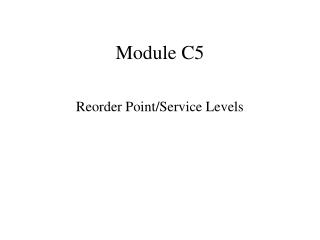DownloadDownload PresentationModule C5

# Module C5

Télécharger la présentation## Module C5

- - - - - - - - - - - - - - - - - - - - - - - - - - - E N D - - - - - - - - - - - - - - - - - - - - - - - - - - -
##### Presentation Transcript

1. Module C5 Reorder Point/Service Levels

2. DETERMINING A REORDER POINT, r* (Without Safety Stock) • Suppose lead time is 8 working days • The company operates 260 days per year • r* = LD where L and D are in the same time units • L = 8/260  .0308 yrs D = 6240 /year r* = .0308(6240)  192 OR, L = 8 days; D/day = 6240/260 = 24 r* = 8(24) = 192

3. DETERMINING A REORDER POINT, r* (With Safety Stock) • Suppose lead time is 8 working days • The company operates 260 days per year • r* = LD + SS • Suppose a safety stock of SS = 13 is desired • L = 8/260  .0308 yrs D = 6240 /year r* = .0308(6240) +13  192 +13 = 205

4. Actual Demand Distribution • Suppose on a short term basis demand actually more closely follows a normal distribution with: • Weekly mean demand W • Weekly variance 2W, Weekly St’d dev. W, • Demand over an n-week period: • normal • Mean nW_ • Variance = n2W, St’d Dev. = (n) W

5. Calculating Q* • Over the course of a year, the standard deviation becomes small relative to the mean value -- hence a common practice is to ignore any variability and calculate Q* by the usual EOQ formula

6. Lead Time Demand • Lead times, however, tend to be short and hence variability must be considered. • A cycle service level is supplied to the modeler -- the probability of not running out of stock during the lead time period. • Suppose lead time is L weeks • Demand during lead time is normal • Mean demand = L = LW • St’d dev. = L = L W

7. Example -- Allen Appliance • Suppose we can assume that demand follows a normal distribution • This can be checked by a “goodness of fit” test • From our data, over the course of a week, W, we can approximate W by (105 + … + 130)/10 = 120 • W2  sW2 = ((1052 +…+1302) - 10(120)2)/9  83.33

8. DEMAND DISTRIBUTION DURING 8 -DAY LEAD TIME • Normal • 8 days = 8/5 = 1.6 weeks, so • L = (1.6)(120) = 192 • L2  (1.6)(83.33) = 133.33 _____ • L 133.33 = 11.55

9. .01 X Z SAFETY STOCK • Suppose we wish a cycle service level of 99% • WE wish NOT to run out of stock in 99% of our inventory cycles L = 11.55 ? 192 0 Z.01 = 2.33

10. Calculating r* and Safety Stock Costs • Reorder point, r* = L + z.01 L= 192 + 2.33(11.55)  219 • Safety stock SS = 2.33(11.55) = 27 • Safety stock cost = ChSS = 1.40(27) = \$37.80 This should be added to the TOTAL ANNUAL COST

11. Reorder Point Enter Lead Time Information Select Cycle Service Level Worksheet Using the Template

12. Module C5 Review • In the short run, demand may seem to follow a probability distribution (normal) • In the long term, variability is relatively insignificant in magnitude compared to the mean value-- so calculate Q* in usual way. • Determine a cycle service level = 1-  • Determine the mean and st’d deviation for demand during lead time • SS = zL r* = L + SS • Safety Stock Costs = ChSS -- add to total cost • Use of Template